### Lane Finding from Video Feeds

February 23, 2017

# Finding Lane Lines on the Road

The github repository with the associate IPython Notebook can be found here.

## Objective

The ultimate goal of this project was to be able to identify lane lines in both images and videos. Namely, to develop a pipeline that utilizes different computer vision techniques to mark the location of lane lines. The approach that I took explored multiple techniques to obtain the best results:

• Color selection with OpenCV with different color maps
• Grayscale
• Hue, saturation, and light
• Gaussian blur to reduce noise
• Canny edge detection
• Hough line transformation
• Moving average of previous lane lines

## Color Manipulation

To start with, images came as screenshots from an onboard video feed.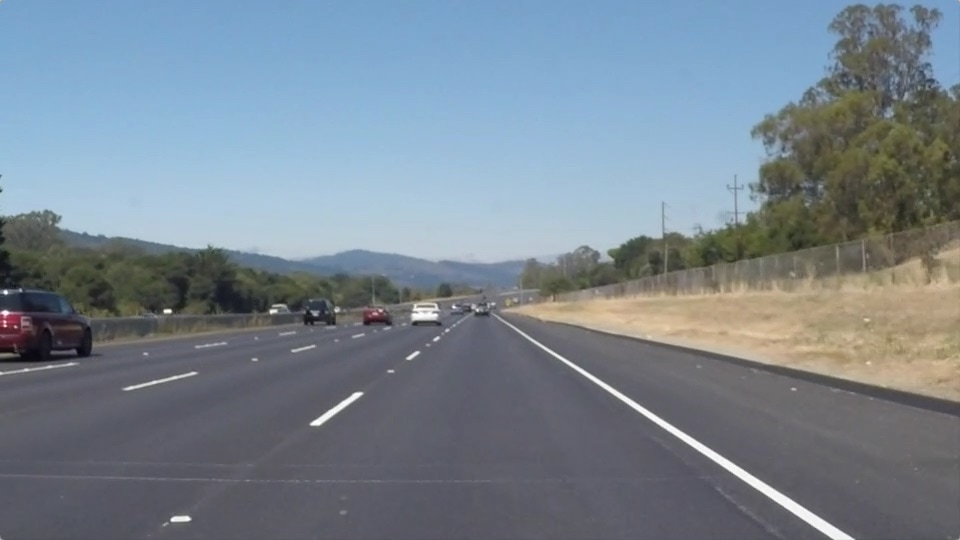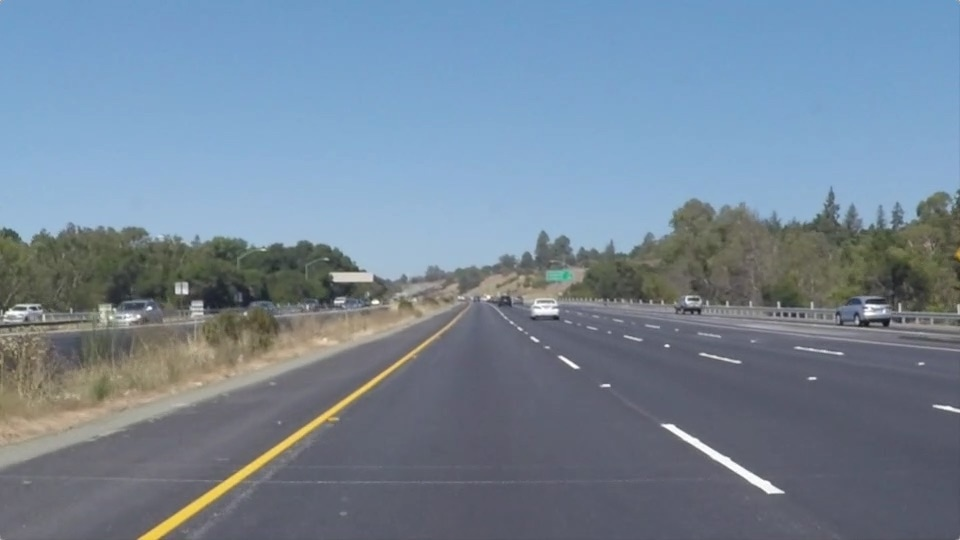The first step that I took was to turn the image to grayscale to make it easier to work, namely to reduce the number of channels to work with. However, when dealing with more challenging images such as lane lines that are on non-contrasting backgrounds (white or gray tarmac), the eventual pipeline for lane linea detection does not perform well. In order to improve the performance, I switched to using hue, saturation, and light color space, which is better able to highlight the yellow and white lane lines.

Grayscale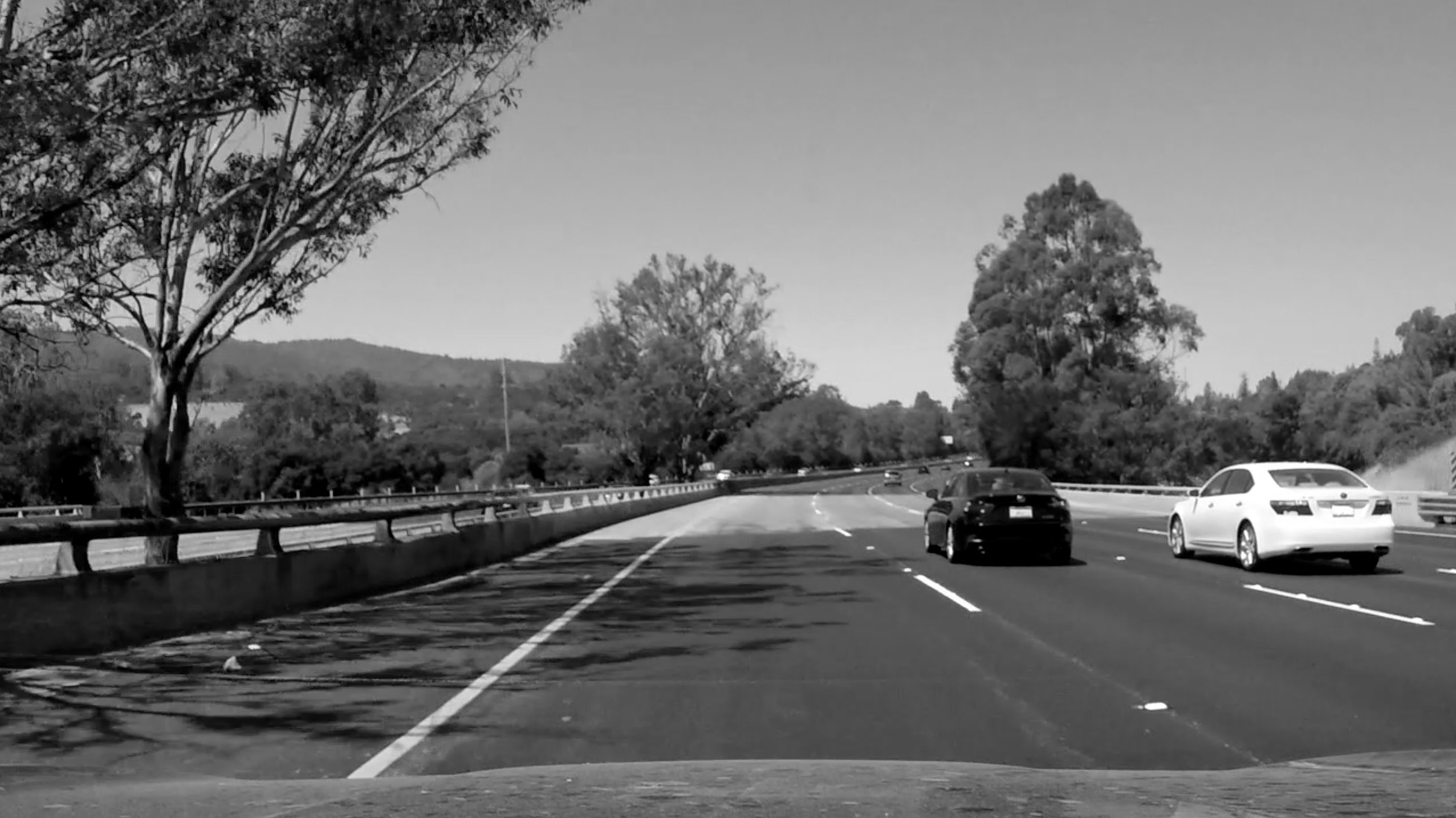HSL color space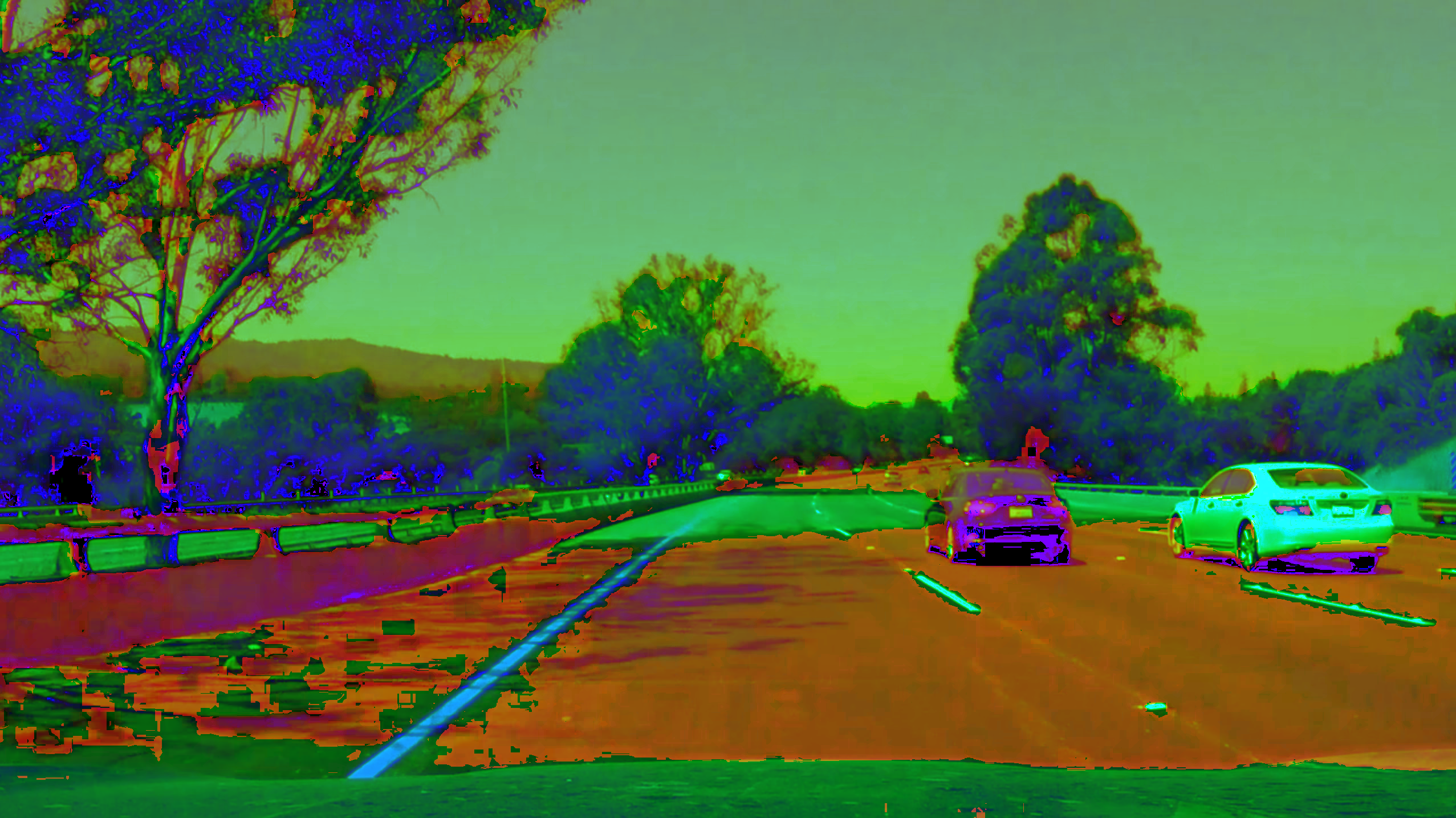In the above image, we can see that the yellow lane is very clearly highlighted and the white line markings are also captured well when compared to the grayscale image. However, to further improve the performance of the processing pipeline, we can also select out the colors that we know we care about (in this case the yellow and white lines, which are now blue and green)

```## color selection for yellow and white, using the HSL color space
def color_selection(image):

hls_image = cv2.cvtColor(image, cv2.COLOR_RGB2HLS)

white_color = cv2.inRange(hls_image, np.uint8([20,200,0]), np.uint8([255,255,255])) ## note that OpenCV uses BGR not RGB
yellow_color = cv2.inRange(hls_image, np.uint8([10,50,100]), np.uint8([100,255,255]))

combined_color_images = cv2.bitwise_or(white_color, yellow_color)
return cv2.bitwise_and(image, image, mask = combined_color_images)
```

In the above code, I first convert the color map from RGB to HSL. Then I use the `inRange` function provided by OpenCV to select colors that fall into the white and yellow ranges. After that I combine the white and yellow masks together with the `bitwise_or` function.

With the above HSL image, we can now try to isolate the yellow and the white lines. While there are many different techniques that can be utilized here, I chose to detect the edges within the image using the Canny edge detection algorithm.

## Edge Detection

HSL color selection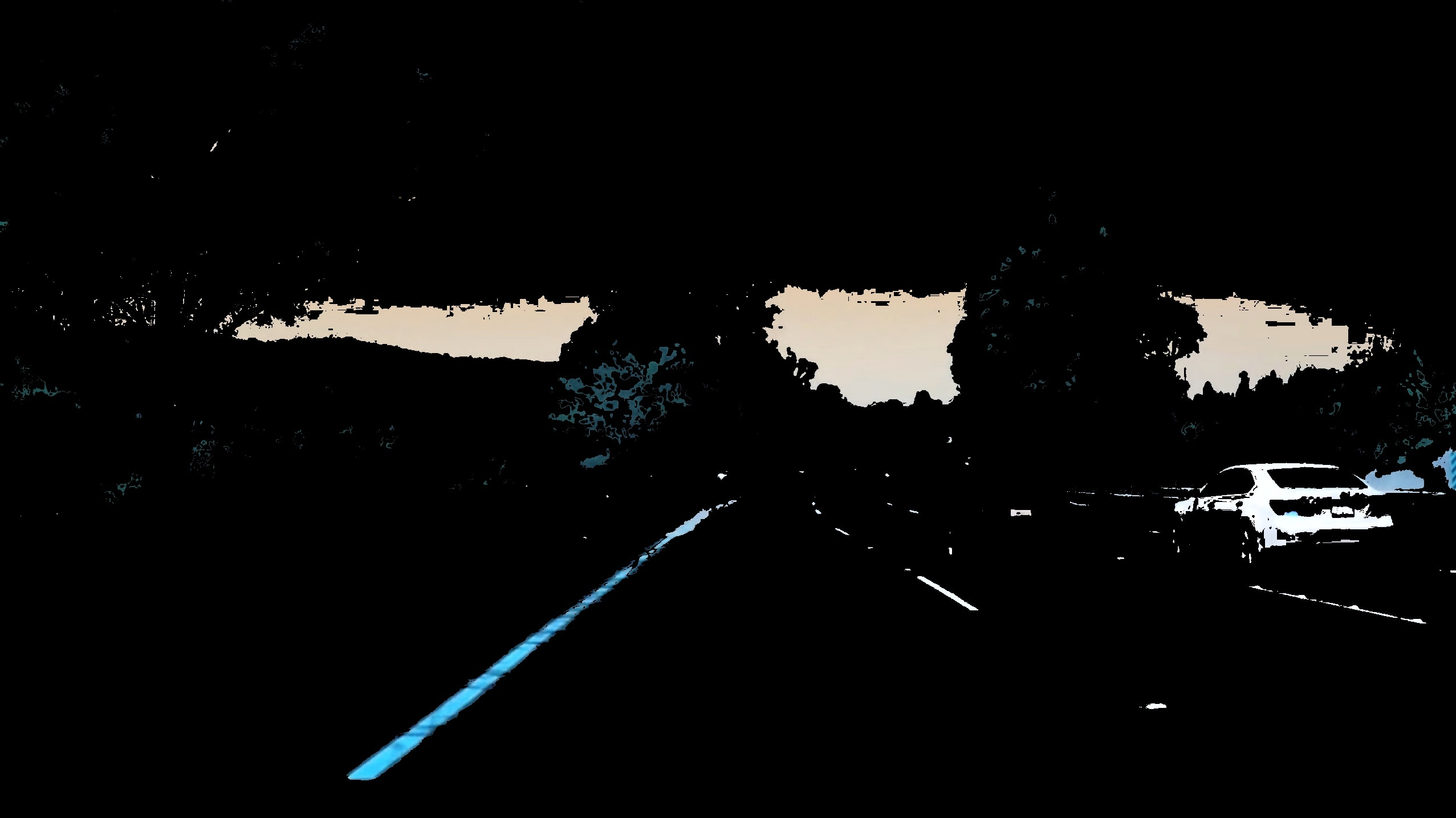Given the above image, the goal is to pick out the lane lines. In order to do this, I use the canny edge detector algorithm. In short, the algorithm:

```1. Applies a gaussian filter to the image to reduce noise
2. Finds the gradients in both the horizontal and vertical directions
3. Non-maximum supression, which is a way to thin the detected edges by only keeping the maximum gradient values and setting others to 0
4. Determining potential edges by checking against a threshold
5. Finish cleaning potential edges by checking in the potential edge is connected to an actual edge
```

While, the canny edge detector automatically applies gaussian blur, I applied gaussian blur outside of the edge detector so that I could have more freedom with the kernel parameter. After running the image through the blurring and edge detection functions, the image is as follows. Note, the input image to this is the HSL color converted image.

HSL color selection with canny edge detection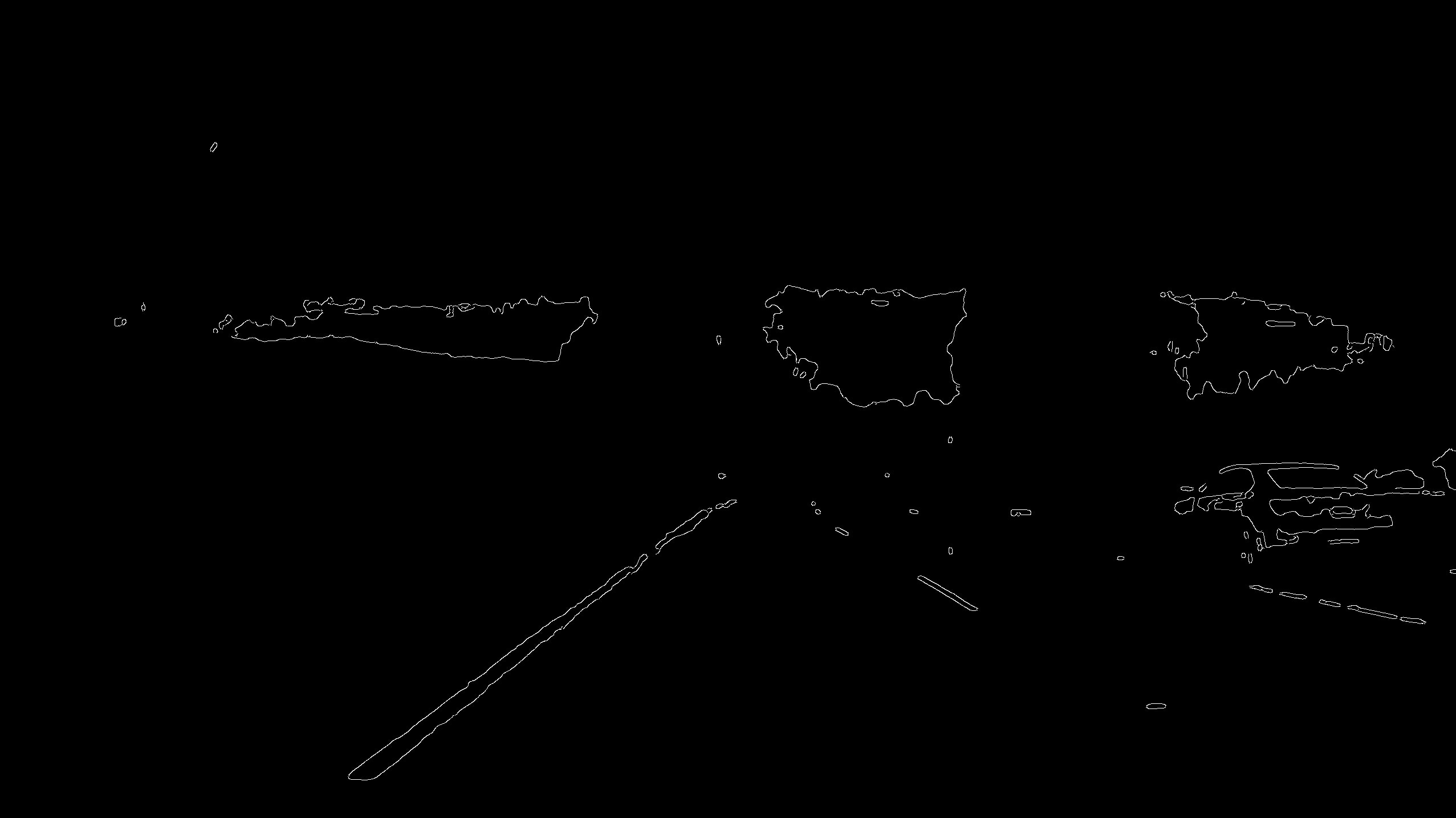With the image above, we see that the lane lines are pretty well identified. It took a bit of trial and error to find suitable thresholds for the canny edge detector though the creator John Canny recommended a ratio of 1:2 or 1:3 for the low vs. high threshold. Although the image above seems to mark the lane lines quite well, there is still a lot of noise surrounding the lane that we do not care about. In order to address this, we can apply a region mask to just keep the area that we know contains the lane lines.

Region masking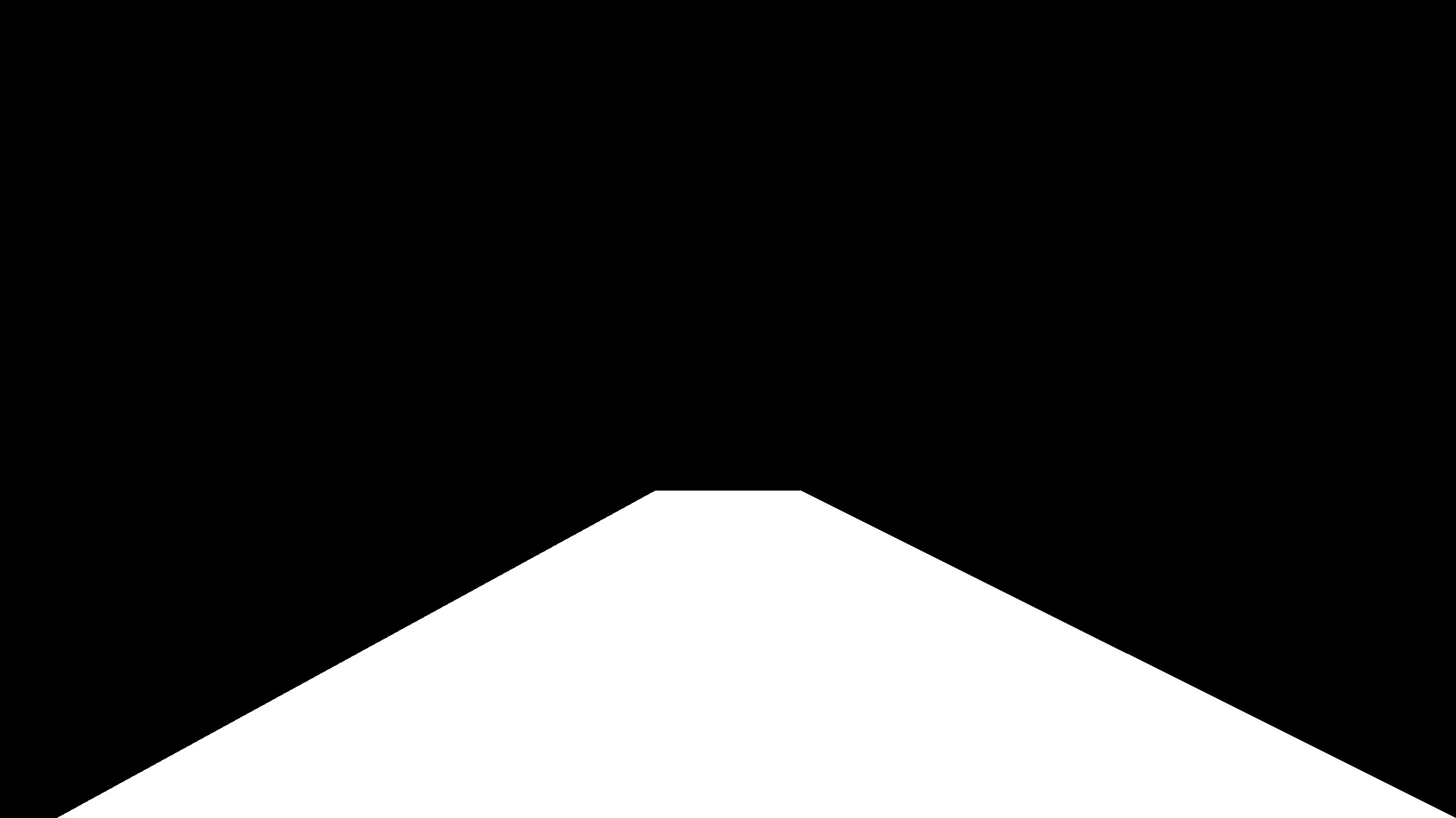After applying the mask to the canny image, we get the following output. We can contrast this with the gray image after canny edge detection and the region selection.

Grayscale image with canny edge detection and region masking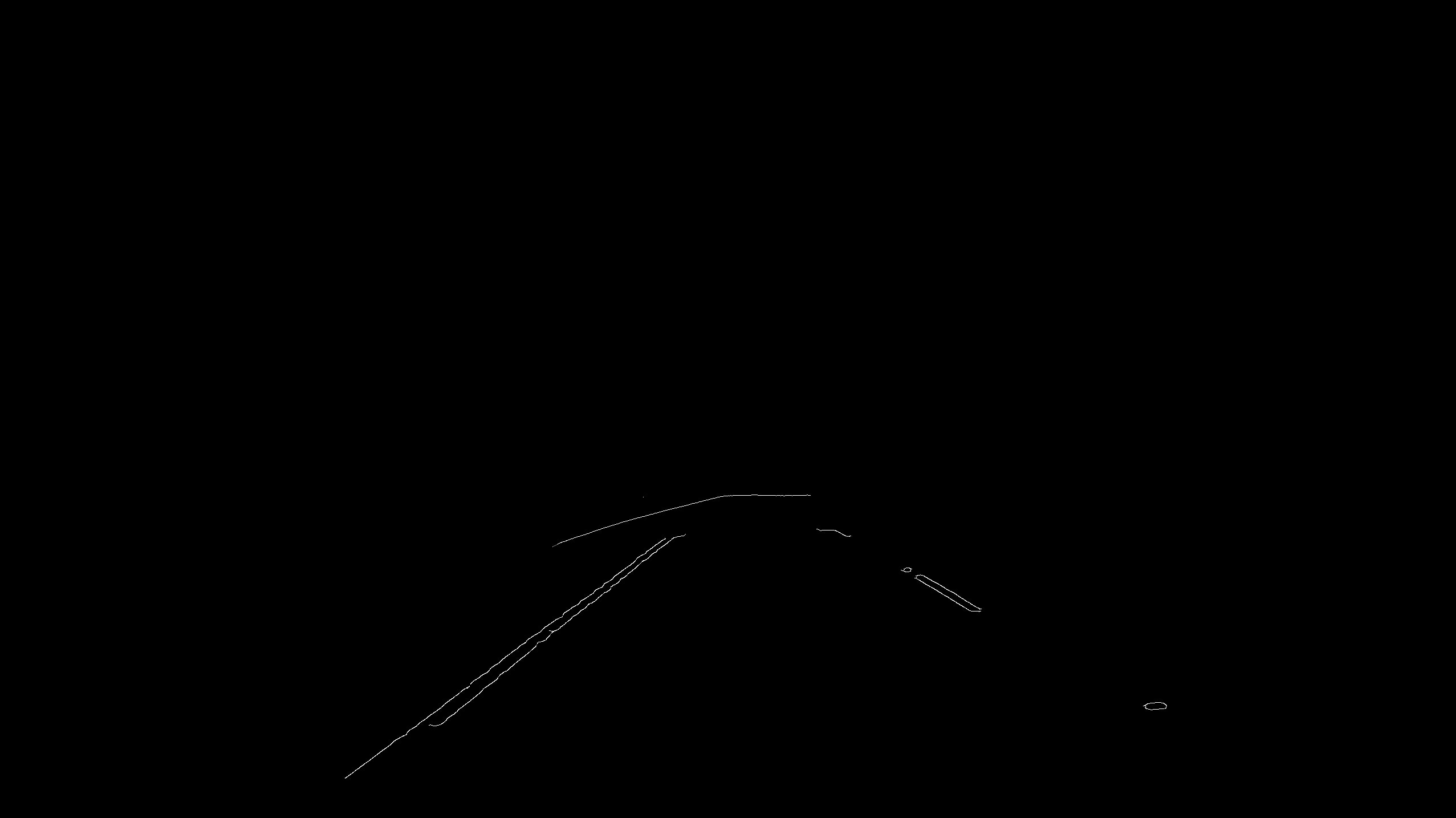HSL color selection with canny edge detection and region masking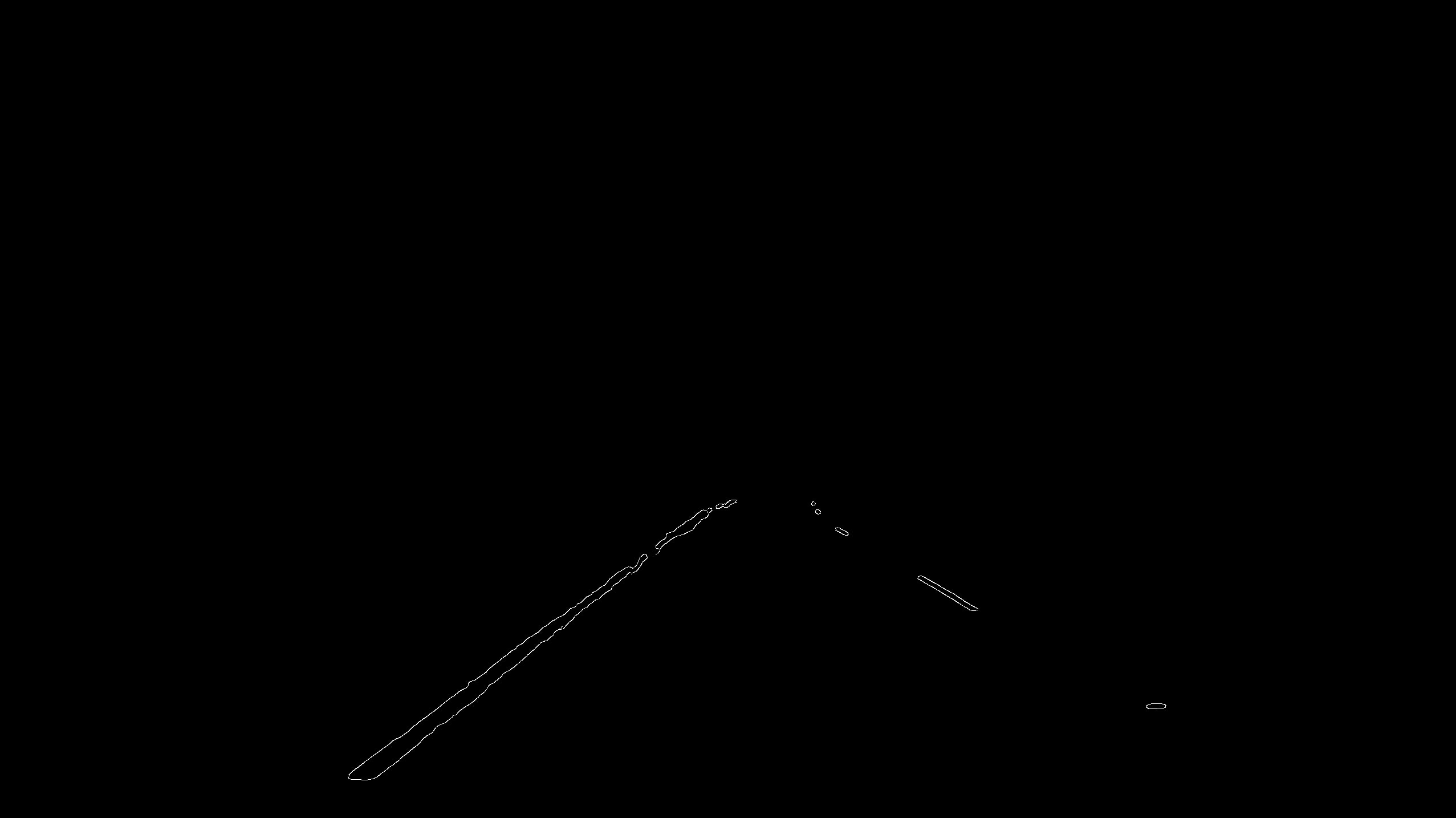As shown above, the HSL version provides a cleaner indication of the lane lines. Below are the functions used in processing the images.

```def canny(img, low_threshold, high_threshold):
"""Applies the Canny transform"""
return cv2.Canny(img, low_threshold, high_threshold)

def gaussian_blur(img, kernel_size):
"""Applies a Gaussian Noise kernel"""
return cv2.GaussianBlur(img, (kernel_size, kernel_size), 0)

def region_of_interest(img, vertices):
"""

Only keeps the region of the image defined by the polygon
formed from `vertices`. The rest of the image is set to black.
"""

#defining a 3 channel or 1 channel color to fill the mask with depending on the input image
if len(img.shape) > 2:
channel_count = img.shape  # i.e. 3 or 4 depending on your image
else:

#filling pixels inside the polygon defined by "vertices" with the fill color

#returning the image only where mask pixels are nonzero
```

## Hough Line Transform

Now that we have a collection of edges, we need to identify the lane lines within the image. The hough line transform, which was first invented to identify lines within images, is great for this task. To learn more about this algorithm, this blog is a great resource.

_HSL color selection with canny edge detection, region masking, and hough transform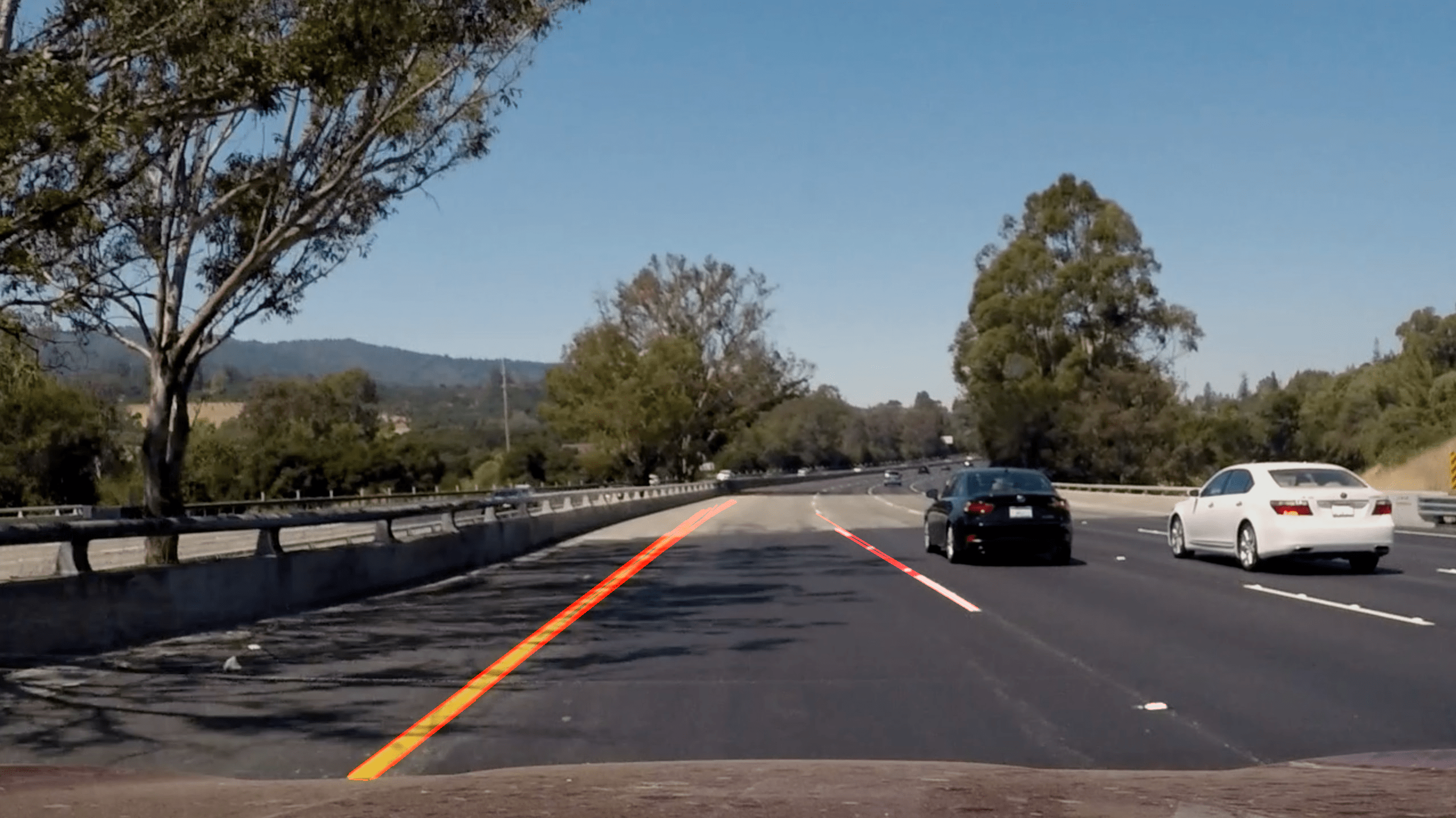Pretty awesome! The lane lines have now been highlighted and boxed with the red lines. There are quite a few parameters that needed to be adjusted, but after adjusting the parameters, the algorithm is able to pick out the lines quite well. Note that the OpenCV version of the hough transform that we are using is the probabilistic version, which is an improvement over the original. In the IPython notebook, I use a different version of the `hough_lines` function that simple outputs the lines as a vector rather than overlaying the lines over the initial image.

Given the above image and specifically the hough lines, we now have a vector of multiple lines segments in the form of (x1,y1,x2,y2) endpoints. In order to draw lines on an image, we need a way to extrapolate an average line from the vector of endpoints.

```def hough_lines_overlay(img, rho, theta, threshold, min_line_len, max_line_gap):
"""
`img` should be the output of a Canny transform.

Returns an image with hough lines drawn.
"""
lines = cv2.HoughLinesP(img, rho, theta, threshold, np.array([]), minLineLength=min_line_len, maxLineGap=max_line_gap)
line_img = np.zeros((img.shape, img.shape, 3), dtype=np.uint8)
draw_lines(line_img, lines)
return line_img
```

## Lane Line Averaging

In order to find the average line on each side of the lane, we can first calculate the slope of each line segment and separate the positive and negative sloped lines, which represents the left and right lane lines. Then we can find the average of the left and right slopes and intercepts to get an average of the lanes. When I initially did this, the average was quite sensitive to outliers. I tried to adjust for the outliers by removing points that were greater than 1.5 standard deviations from the rest of the slopes. However, the averaged lines were still quite sensitive to the outliers.

Ultimately, by calculating the line length and calculating the weighted average of the lane line, the output was much more stable and robust against spurious line segments that the hough transform identified.

```def avg_lines(lines):

neg = np.empty([1,3])
pos = np.empty([1,3])

## calculate slopes for each line to identify the positive and negative lines
for line in lines:
for x1,y1,x2,y2 in line:
slope = (y2-y1)/(x2-x1)
intercept = y1 - slope*x1
line_length = np.sqrt((y2-y1)**2+(x2-x1)**2)
if slope < 0 and line_length > 10:
neg = np.append(neg,np.array([[slope, intercept, line_length]]),axis = 0)
elif slope > 0 and line_length > 10:
pos = np.append(pos,np.array([[slope, intercept, line_length]]),axis = 0)

## just keep the observations with slopes with 2 std dev
neg = neg[to_keep_index(neg[:,0])]
pos = pos[to_keep_index(pos[:,0])]

## weighted average of the slopes and intercepts based on the length of the line segment
neg_lines = np.dot(neg[1:,2],neg[1:,:2])/np.sum(neg[1:,2]) if len(neg[1:,2]) > 0 else None
pos_lines = np.dot(pos[1:,2],pos[1:,:2])/np.sum(pos[1:,2]) if len(pos[1:,2]) > 0 else None

return neg_lines, pos_lines

## removing the outliers (adapted from http://stackoverflow.com/questions/11686720/is-there-a-numpy-builtin-to-reject-outliers-from-a-list)
def to_keep_index(obs, std=1.5):
return np.array(abs(obs - np.mean(obs)) < std*np.std(obs))
```

The above function calculates the slope, intercept, and line length of each line segment. At this point, we can take the average lane lines from the above function and plot the lane lines onto the original image.

Final processed image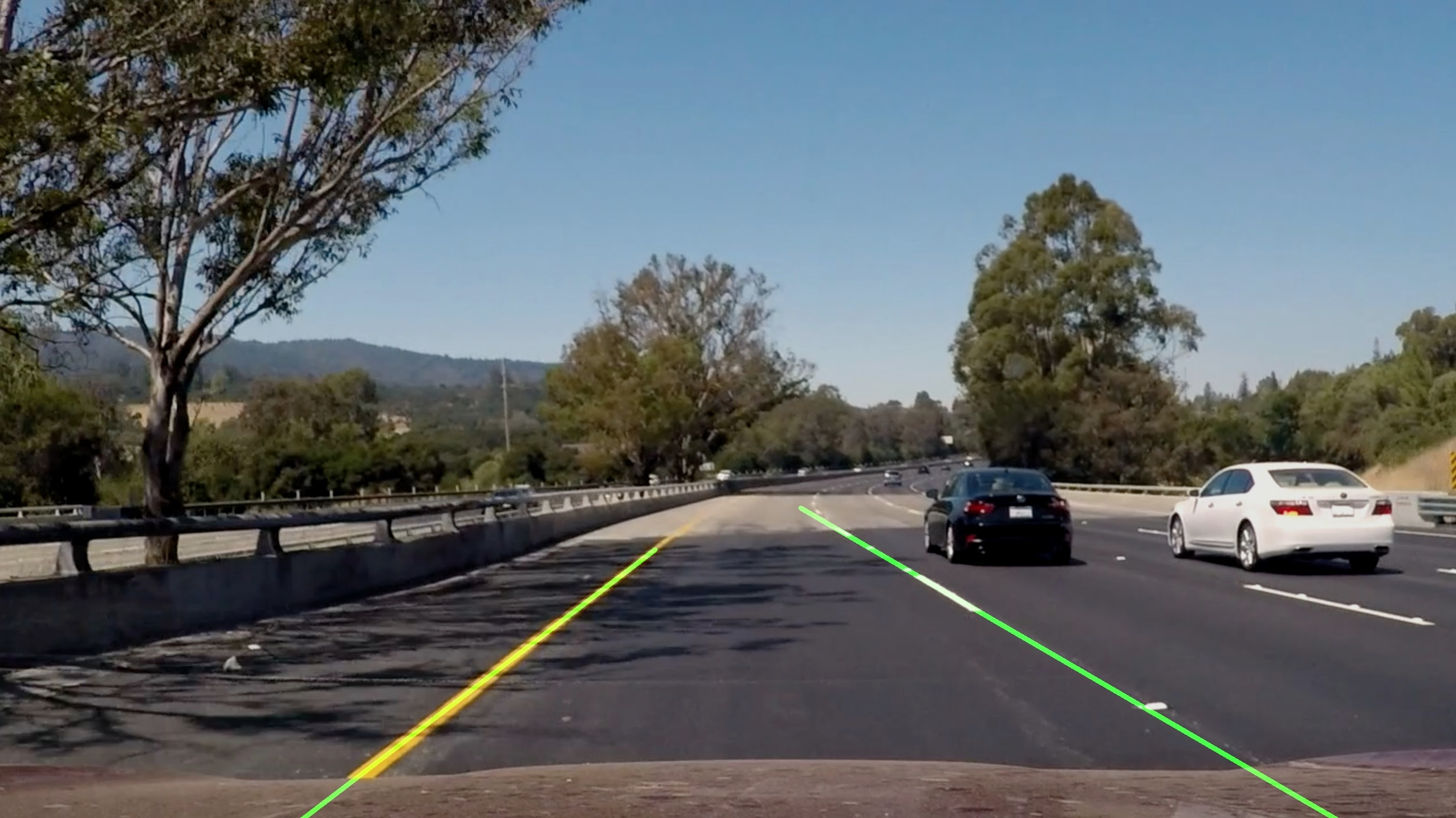It seems to have performed quite well! Below are a few other sample images of the outputs from the lane finding pipeline.

Sample processed images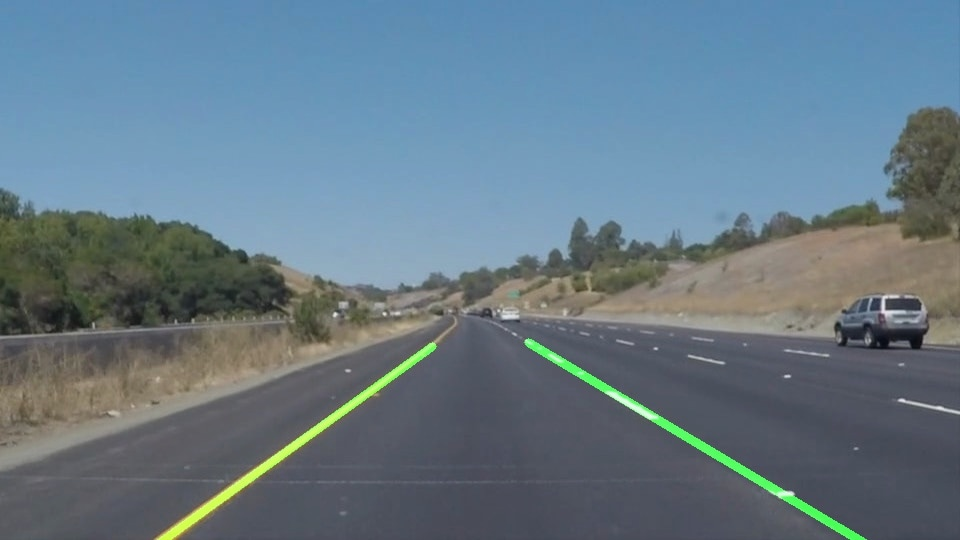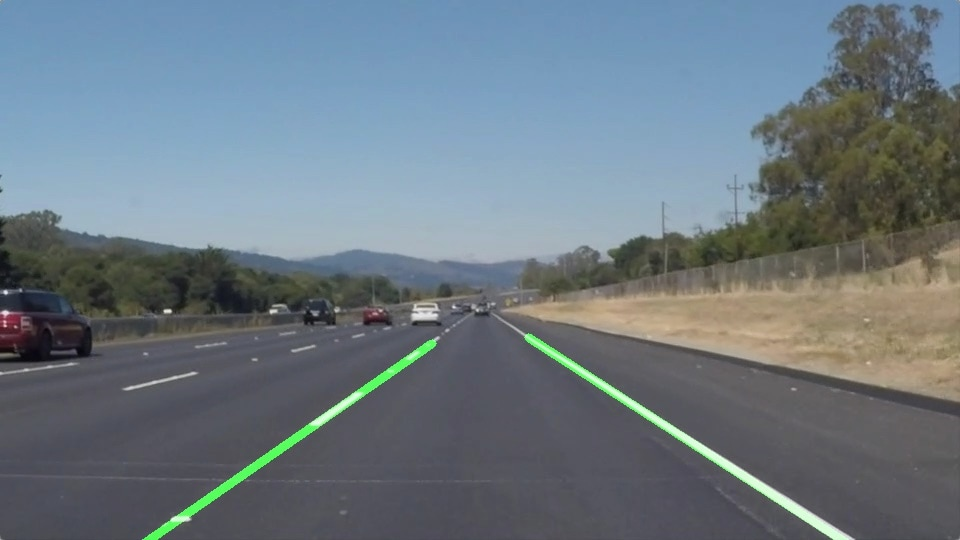## Applying Lane Finding to Videos

Now that we can identify and mark the lane lines within the image supplied, we can use the algorithm on a video, which is just a sequence of images. If we just apply the pipeline directly to the video, we get the following.

The video seems to show the lane lines without any problems, but when we take a closer look the lane line highlights are jittering and jumping across back and forth around the actual location of the lane line. While, the algorithm basically accomplishes the problem that we first set out to solve, maybe we can improve on this.

Specifically, the lane lines coming from a video feed usually do not change dramatically from second to second. If we take this into account, we can "smooth" the lane lines plotted out by keeping a queue. With each frame of the video, we can pop off the oldest set of lane line endpoints. Then for all the remaining lane lines and the newest lane line, we take an average to get the "smoothed" lane line.

Below is the code for the lane line detector and the link to the test videos.

The above videos show that the new detector with the lane line averaging works quite nicely! Although if there are drastic changes the algorithm does not follow those changes until a bit later, we can fiddle with this by changing the size of the queue.

```class lane_detector:
def __init__(self):
self.prev_lane_lines = []

def find_mean_lines(self, lane_lines, prev_lane_lines):

## add the new lane line
if lane_lines is not None:
prev_lane_lines.append(lane_lines)

## only keep the 10 most recent lane lines
if len(prev_lane_lines) >= 10:
prev_lane_lines.pop(0)

## take the average of the past lane lines and the new ones
if len(prev_lane_lines) > 0:
return np.mean(prev_lane_lines, axis = 0, dtype=np.int)

def pipeline(self, image):
imshape = image.shape

## selecting yellow and white colors
color_selected_img = color_selection(image)

## Define a kernel size and apply Gaussian smoothing
kernel_size = 17
blur_img = cv2.GaussianBlur(color_selected_img,(kernel_size, kernel_size),0)

## apply canny edge detection
canny_img = canny(blur_img, low_threshold= 50, high_threshold=160)

## apply region of interest
vertices = np.array([[(100,imshape),(imshape*.45, imshape*0.6), (imshape*.55, imshape*0.6), (imshape,imshape)]], dtype=np.int32)
region_img = region_of_interest(canny_img, vertices=vertices)

## apply hough transformation
rho = 1 # distance resolution in pixels of the Hough grid
theta = np.pi/180 # angular resolution in radians of the Hough grid
threshold = 15 # minimum number of votes (intersections in Hough grid cell)
min_line_len = 25 #minimum number of pixels making up a line
max_line_gap = 250   # maximum gap in pixels between connectable line segments

lines = hough_lines(region_img, rho, theta, threshold, min_line_len, max_line_gap)

## get the average slopes and intercepts for each lane line
slopes_intercepts = avg_lines(lines)

# find the endpoints given the slopes and intercepts
endpoints = gen_endpoints(image, slopes_intercepts)

## generate lane lines on a black image
lane_lines = gen_lane_lines(image, endpoints=self.find_mean_lines(endpoints, self.prev_lane_lines))

final_img = weighted_img(lane_lines, image)

return final_img
```

## Shortcomings & Next Steps

While the detector works fairly well for straight roads, there are limitations: